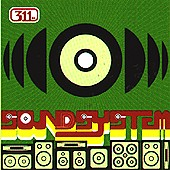# 311

This number is a prime.

Just showing those entries submitted by 'Loungrides': (Click here to show all)The only home emirp for two distinct composite numbers n <100, (cases n=9 and n=33). [Loungrides]The only prime (emirp) that can be represented as p p’s minus q q’s, where p, q are successive primes, i.e., 333-22. [Loungrides]The smallest prime (emirp) that is the first of six consecutive primes that remain prime if you delete their first digit, i.e., 311, 313, 317, 331, 337, 347. [Loungrides]The smallest emirp that can be represented as sum of the four powers a^a+b^b+c^c+d^d where abcd is a 4-digit prime p, i.e., (p=1433), with 1^1+4^4+3^3+3^3=311. [Loungrides]